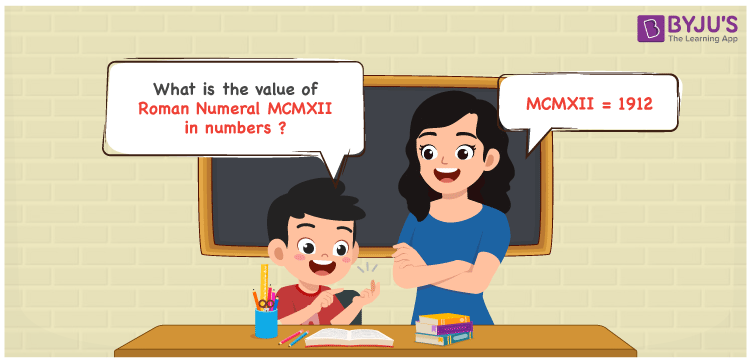Checkout JEE MAINS 2022 Question Paper Analysis : Checkout JEE MAINS 2022 Question Paper Analysis :

# MCMXII Roman Numerals

MCMXII Roman Numerals is 1912. Roman Numerals are the way of expressing numbers using the Roman alphabet. Access the PDF of Roman Numerals Conversion to learn the simple tricks of expressing Roman letters into numbers in a precise manner. In this article, we will learn how to represent the value of MCMXII Roman Numerals in numbers with a complete explanation.

 Number Roman Numeral 1912 MCMXII

## How to Write MCMXII Roman Numerals?The value of MCMXII Roman Numerals can be expressed by using any of the two methods given below

First Method:

• Break the Roman Numerals into single letters
• MCMXII = M + (M – C) + X + I + I
• Write the numerical value of each letter and add/subtract them
• MCMXII = 1000 + (1000 – 100) + 10 + 1 + 1 = 1912

Second Method:

In this method, we consider the groups of Roman Numerals for addition or subtraction

• MCMXII = M + CM + X + II = 1000 + 900 + 10 + 2 = 1912
• Hence the numerical value of MCMXII Roman Numerals is 1912

## Video Lesson on Roman Numerals## Related Articles

Roman Numerals

Roman Numerals 1 to 50

Roman Numerals 1 to 100

Roman Numerals 1 to 1000

L Roman Numerals

Roman Numeral XXXIX

## Frequently Asked Questions on MCMXII Roman Numerals

### What is MCMXII Roman Numerals?

MCMXII Roman Numerals is 1912.

### What is the value of 1800 + 112 in Roman Numerals?

1800 + 112 = 1912. The number 1912 in Roman Numerals is MCMXII. Hence the value of 1800 + 112 in Roman Numerals is MCMXII.

### How do you read the Roman Numeral MCMXII in English?

MCMXII Roman Numeral is equal to 1912 and it is read as One Thousand Nine Hundred Twelve in English.